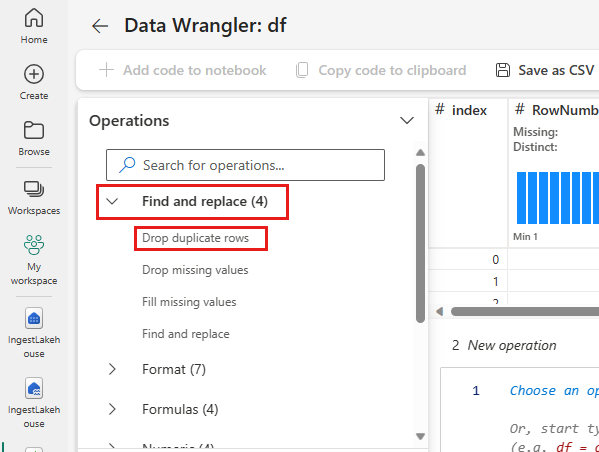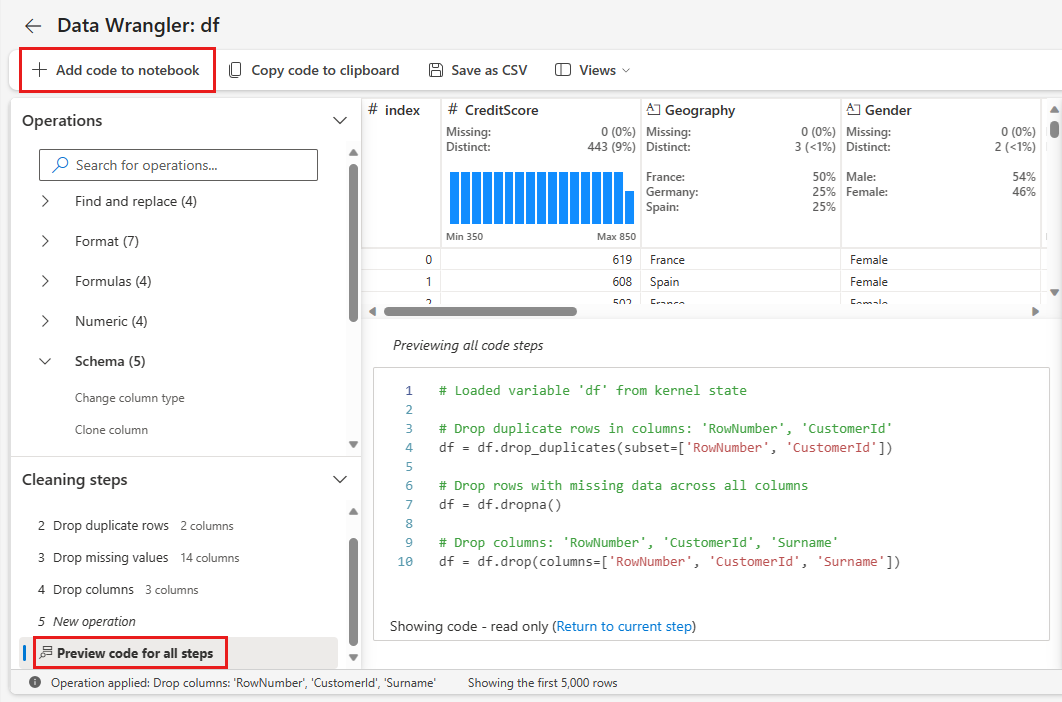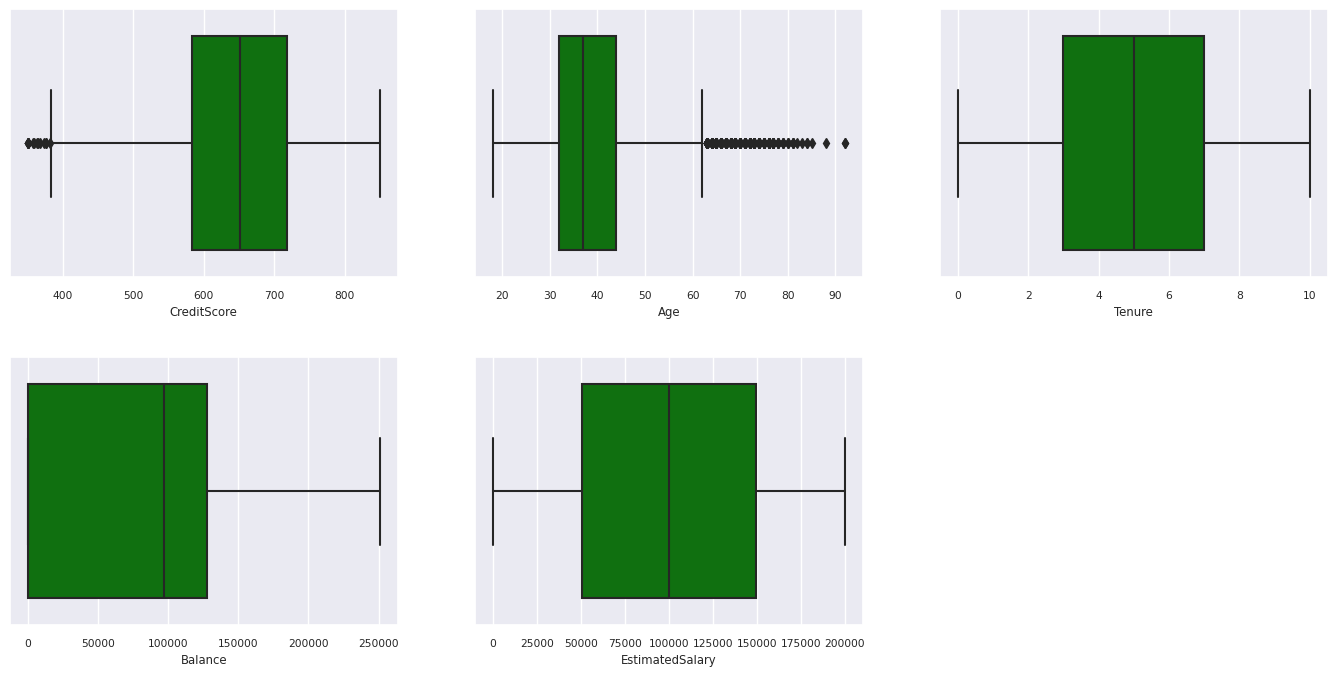# Tutorial Part 2: Explore and visualize data using Microsoft Fabric notebooks

In this tutorial, you'll learn how to conduct exploratory data analysis (EDA) to examine and investigate the data while summarizing its key characteristics through the use of data visualization techniques.

You'll use `seaborn`, a Python data visualization library that provides a high-level interface for building visuals on dataframes and arrays. For more information about `seaborn`, see Seaborn: Statistical Data Visualization.

You'll also use Data Wrangler, a notebook-based tool that provides you with an immersive experience to conduct exploratory data analysis and cleaning.

The main steps in this tutorial are:

1. Read the data stored from a delta table in the lakehouse.
2. Convert a Spark DataFrame to Pandas DataFrame, which python visualization libraries support.
3. Use Data Wrangler to perform initial data cleaning and transformation.
4. Perform exploratory data analysis using `seaborn`.

## Prerequisites

This is part 2 of 5 in the tutorial series. To complete this tutorial, first complete:

2-explore-cleanse-data.ipynb is the notebook that accompanies this tutorial.

If you want to open the accompanying notebook for this tutorial, follow the instructions in Prepare your system for data science to import the tutorial notebooks to your workspace.

Or, if you'd rather copy and paste the code from this page, you can create a new notebook.

Be sure to attach a lakehouse to the notebook before you start running code.

Important

Attach the same lakehouse you used in Part 1.

## Read raw data from the lakehouse

Read raw data from the Files section of the lakehouse. You uploaded this data in the previous notebook. Make sure you have attached the same lakehouse you used in Part 1 to this notebook before you run this code.

``````df = (
.option("inferSchema", True)
.csv("Files/churn/raw/churn.csv")
.cache()
)
``````

## Create a pandas DataFrame from the dataset

Convert the spark DataFrame to pandas DataFrame for easier processing and visualization.

``````df = df.toPandas()
``````

### Display raw data

Explore the raw data with `display`, do some basic statistics and show chart views. Note that you first need to import the required libraries such as `Numpy`, `Pnadas`, `Seaborn`, and `Matplotlib` for data analysis and visualization.

``````import seaborn as sns
sns.set_theme(style="whitegrid", palette="tab10", rc = {'figure.figsize':(9,6)})
import matplotlib.pyplot as plt
import matplotlib.ticker as mticker
from matplotlib import rc, rcParams
import numpy as np
import pandas as pd
import itertools
``````
``````display(df, summary=True)
``````

## Use Data Wrangler to perform initial data cleaning

To explore and transform any pandas Dataframes in your notebook, launch Data Wrangler directly from the notebook.

Note

Data Wrangler can not be opened while the notebook kernel is busy. The cell execution must complete prior to launching Data Wrangler.

1. Under the notebook ribbon Data tab, select Launch Data Wrangler. You'll see a list of activated pandas DataFrames available for editing.
2. Select the DataFrame you wish to open in Data Wrangler. Since this notebook only contains one DataFrame, `pd`, select `pd`.Data Wrangler launches and generates a descriptive overview of your data. The table in the middle shows each data column. The Summary panel next to the table shows information about the DataFrame. When you select a column in the table, the summary updates with information about the selected column. In some instances, the data displayed and summarized will be a truncated view of your DataFrame. When this happens, you'll see warning image in the summary pane. Hover over this warning to view text explaining the situation.

Each operation you do can be applied in a matter of clicks, updating the data display in real time and generating code that you can save back to your notebook as a reusable function.

The rest of this section walks you through the steps to perform data cleaning with Data Wrangler.

## Drop duplicate rows

On the left panel is a list of operations (such as Find and replace, Format, Formulas, Numeric) you can perform on the dataset.

1. Expand Find and replace and select Drop duplicate rows.2. A panel appears for you to select the list of columns you want to compare to define a duplicate row. Select RowNumber and CustomerId.

In the middle panel is a preview of the results of this operation. Under the preview is the code to perform the operation. In this instance, the data appears to be unchanged. But since you're looking at a truncated view, it's a good idea to still apply the operation.

3. Select Apply (either at the side or at the bottom) to go to the next step.

### Drop rows with missing data

Use Data Wrangler to drop rows with missing data across all columns.

1. Select Drop missing values from Find and replace.

2. Select All columns from the drop-down list.

3. Select Apply to go on to the next step.

### Drop columns

Use Data Wrangler to drop columns that you don't need.

1. Expand Schema and select Drop columns.

2. Select RowNumber, CustomerId, Surname. These columns appear in red in the preview, to show they're changed by the code (in this case, dropped.)

3. Select Apply to go on to the next step.

Each time you select Apply, a new step is created in the Cleaning steps panel on the bottom left. At the bottom of the panel, select Preview code for all steps to view a combination of all the separate steps.

Select Add code to notebook at the top left to close Data Wrangler and add the code automatically. The Add code to notebook wraps the code in a function, then calls the function.Tip

The code generated by Data Wrangler won't be applied until you manually run the new cell.

If you didn't use Data Wrangler, you can instead use this next code cell.

This code is similar to the code produced by Data Wrangler, but adds in the argument `inplace=True` to each of the generated steps. By setting `inplace=True`, pandas will overwrite the original DataFrame instead of producing a new DataFrame as an output.

``````# Modified version of code generated by Data Wrangler
# Modification is to add in-place=True to each step

# Define a new function that include all above Data Wrangler operations
def clean_data(df):
# Drop rows with missing data across all columns
df.dropna(inplace=True)
# Drop duplicate rows in columns: 'RowNumber', 'CustomerId'
df.drop_duplicates(subset=['RowNumber', 'CustomerId'], inplace=True)
# Drop columns: 'RowNumber', 'CustomerId', 'Surname'
df.drop(columns=['RowNumber', 'CustomerId', 'Surname'], inplace=True)
return df

df_clean = clean_data(df.copy())
``````

## Explore the data

Display some summaries and visualizations of the cleaned data.

### Determine categorical, numerical, and target attributes

Use this code to determine categorical, numerical, and target attributes.

``````# Determine the dependent (target) attribute
dependent_variable_name = "Exited"
print(dependent_variable_name)
# Determine the categorical attributes
categorical_variables = [col for col in df_clean.columns if col in "O"
or df_clean[col].nunique() <=5
and col not in "Exited"]
print(categorical_variables)
# Determine the numerical attributes
numeric_variables = [col for col in df_clean.columns if df_clean[col].dtype != "object"
and df_clean[col].nunique() >5]
print(numeric_variables)
``````

### The five-number summary

Show the five-number summary (the minimum score, first quartile, median, third quartile, the maximum score) for the numerical attributes, using box plots.

``````df_num_cols = df_clean[numeric_variables]
sns.set(font_scale = 0.7)
fig, axes = plt.subplots(nrows = 2, ncols = 3, gridspec_kw =  dict(hspace=0.3), figsize = (17,8))
fig.tight_layout()
for ax,col in zip(axes.flatten(), df_num_cols.columns):
sns.boxplot(x = df_num_cols[col], color='green', ax = ax)
fig.delaxes(axes[1,2])

``````## Distribution of exited and nonexited customers

Show the distribution of exited versus nonexited customers across the categorical attributes.

``````attr_list = ['Geography', 'Gender', 'HasCrCard', 'IsActiveMember', 'NumOfProducts', 'Tenure']
fig, axarr = plt.subplots(2, 3, figsize=(15, 4))
for ind, item in enumerate (attr_list):
sns.countplot(x = item, hue = 'Exited', data = df_clean, ax = axarr[ind%2][ind//2])
``````

### Distribution of numerical attributes

Show the frequency distribution of numerical attributes using histogram.

``````columns = df_num_cols.columns[: len(df_num_cols.columns)]
fig = plt.figure()
fig.set_size_inches(18, 8)
length = len(columns)
for i,j in itertools.zip_longest(columns, range(length)):
plt.subplot((length // 2), 3, j+1)
plt.subplots_adjust(wspace = 0.2, hspace = 0.5)
df_num_cols[i].hist(bins = 20, edgecolor = 'black')
plt.title(i)
plt.show()
``````

## Perform feature engineering

Perform feature engineering to generate new attributes based on current attributes:

``````df_clean["NewTenure"] = df_clean["Tenure"]/df_clean["Age"]
df_clean["NewCreditsScore"] = pd.qcut(df_clean['CreditScore'], 6, labels = [1, 2, 3, 4, 5, 6])
df_clean["NewAgeScore"] = pd.qcut(df_clean['Age'], 8, labels = [1, 2, 3, 4, 5, 6, 7, 8])
df_clean["NewBalanceScore"] = pd.qcut(df_clean['Balance'].rank(method="first"), 5, labels = [1, 2, 3, 4, 5])
df_clean["NewEstSalaryScore"] = pd.qcut(df_clean['EstimatedSalary'], 10, labels = [1, 2, 3, 4, 5, 6, 7, 8, 9, 10])
``````

## Use Data Wrangler to perform one-hot encoding

Data Wrangler can also be used to perform one-hot encoding. To do so, re-open Data Wrangler. This time, select the `df_clean` data.

1. Expand Formulas and select One-hot encode.
2. A panel appears for you to select the list of columns you want to perform one-hot encoding on. Select Geography and Gender.

You could copy the generated code, close Data Wrangler to return to the notebook, then paste into a new cell. Or, select Add code to notebook at the top left to close Data Wrangler and add the code automatically.

If you didn't use Data Wrangler, you can instead use this next code cell:

``````# This is the same code that Data Wrangler will generate

import pandas as pd

def clean_data(df_clean):
# One-hot encode columns: 'Geography', 'Gender'
df_clean = pd.get_dummies(df_clean, columns=['Geography', 'Gender'])
return df_clean

df_clean_1 = clean_data(df_clean.copy())
``````

## Summary of observations from the exploratory data analysis

• Most of the customers are from France comparing to Spain and Germany, while Spain has the lowest churn rate comparing to France and Germany.
• Most of the customers have credit cards.
• There are customers whose age and credit score are above 60 and below 400, respectively, but they can't be considered as outliers.
• Very few customers have more than two of the bank's products.
• Customers who aren't active have a higher churn rate.
• Gender and tenure years don't seem to have an impact on customer's decision to close the bank account.

## Create a delta table for the cleaned data

You'll use this data in the next notebook of this series.

``````table_name = "df_clean"
# Create Spark DataFrame from pandas
sparkDF=spark.createDataFrame(df_clean_1)
sparkDF.write.mode("overwrite").format("delta").save(f"Tables/{table_name}")
print(f"Spark dataframe saved to delta table: {table_name}")
``````

## Next step

Train and register machine learning models with this data: